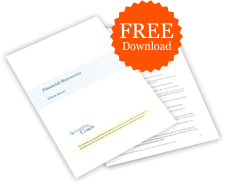# What is financial leverage?

## Definition of Financial Leverage

Financial leverage which is also known as leverage or trading on equity, refers to the use of debt to acquire additional assets.

The use of financial leverage to control a greater amount of assets (by borrowing money) will cause the returns on the owner's cash investment to be amplified. That is, with financial leverage:

• an increase in the value of the assets will result in a larger gain on the owner's cash, when the loan interest rate is less than the rate of increase in the asset's value
• a decrease in the value of the assets will result in a larger loss on the owner's cash

## Examples of Financial Leverage

Mary uses \$500,000 of her cash to purchase 40 acres of land with a total cost of \$500,000. Mary is not using financial leverage.

Sue uses \$500,000 of her cash and borrows \$1,000,000 to purchase 120 acres of land having a total cost of \$1,500,000. Sue is using financial leverage to own/control \$1,500,000 of property with only \$500,000 of her own money. Let's also assume that the interest on Sue's loan is \$50,000 per year and it is paid at the beginning of each year.

## Effects of Using Financial Leverage

For our examples let's assume that after one year, the land owned by Mary and the land owned by Sue increased in value by 20% and both Mary and Sue sold their land investments at the market values. As a result:

• Mary's land will sell for \$600,000 which results in a gain of \$100,000 (selling price of \$600,000 minus the land's cost of \$500,000). The \$100,000 gain on Mary's cash of \$500,000 results in a gain of 20% on Mary's \$100,000
• Sue's land will sell for \$1,800,000 which results in a gain of \$250,000 (selling price of \$1,800,000 minus \$1,550,000, which is the land's cost of \$1,500,000 and interest of \$50,000). The \$250,000 gain on Sue's \$550,000 of cash is a gain of 45% instead of a gain of 20% without the use of leverage

Now let's assume that after one year, the land owned by Mary and and the land owned by Sue decreased in value by 20% and that both Mary and Sue sold their land investments at the market values. As a result:

• Mary's land will sell for \$400,000 which means a \$100,000 loss on the land's cost of \$500,000. The \$100,000 loss on Mary's cash of \$500,000 results in a 20% loss on Mary's money
• Sue's land will sell for \$1,200,000 which results in a loss of \$350,000 (selling price of \$1,200,000 minus the land's cost of \$1,500,000 and interest of \$50,000). The \$350,000 loss on Sue's cash of \$550,000 results in a 63.6% loss on Sue's money instead of a loss of 20% without the use of leverage### Free Financial Statements Cheat Sheet

543,653
Subscribers
You are already subscribed. This offer is not available to existing subscribers.
Error: You have unsubscribed from this list.